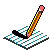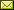PHYSICS@HORTONPhysics12

Physics 11

Math 11

NS Exams

Curriculum

Tips

Tutorials

McGraw Hill

Resources

Need it?

News

Enrichment

GiancoliIB Physics SL

April 2017 Notes

 April 3 PRESCRIBED PRACTICAL ~ Measuring the acceleration due to gravity by timing a freefalling object (write up as DCP/CE) Try the following simulation: Free Fall Model April 4 work on LABS, Popper Physics, Reaction Time April 5 work on LABS, Popper Physics, Reaction Time April 6 QUIZ (2.1 Kinematics) work on Labs April 7 Motion on an Incline April 10 April 11 Forces (Weight/Normal Force/FBDs) BrainPop: Gravity April 12 Forces (Resultant Forces/Balanced & Unbalanced Forces/Newton's First Law/Translational Equilibrium/Newton's Second Law) Notes April 13 Forces (Newton's Second Law) BrainPop: Force Forces & Motion: Basics (Tug of War & Acceleration Lab) (needs Java) Negative Mass?? Notes April 18 Forces (Friction) The Physics Classroom The Calculator Pad Forces (Fluid Resistance) Notes April 19 Investigating the Terminal Velocity of a Coffee Filter April 20 Work on Terminal Velocity Lab April 21 Forces in 1D (needs Java) Work on "TO DO" list April 24 Momentum & Impulse (Momentum, Impulse & Newton's Second Law/F-t graphs/Conservation of Linear Momentum) Momentum & Impulse More Momentum & Impulse Impulse Bouncing Ball Momentum & Collisions (PHYSCLIPS) Notes April 25 Momentum & Impulse (Conservation of Linear Momentum) Finish Popper Physics pHET Collision Lab (needs JAVA!!! ~ settings: 1D, check velocity vectors & momenta diagram, try 100%, 50%, 0% elasticity, try different mass combinations, try different velocities and look at the total mometum before & after collision) Notes April 26 Momentum & Impulse (Conservation of Linear Momentum) Cannon Ball Fired from Back of Moving Truck Notes Momentum & Impulse (Conservation of Linear Momentum) Notes Video: Momentum (Vector Addition) 2D Collisions (World-in-Motion video analysis) Another Example April 27 Momentum & Impulse (Newton's Third Law) Notes

{subdirectory}